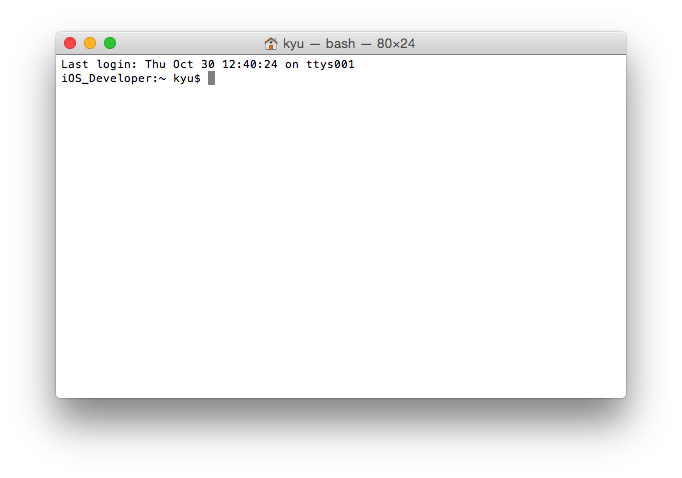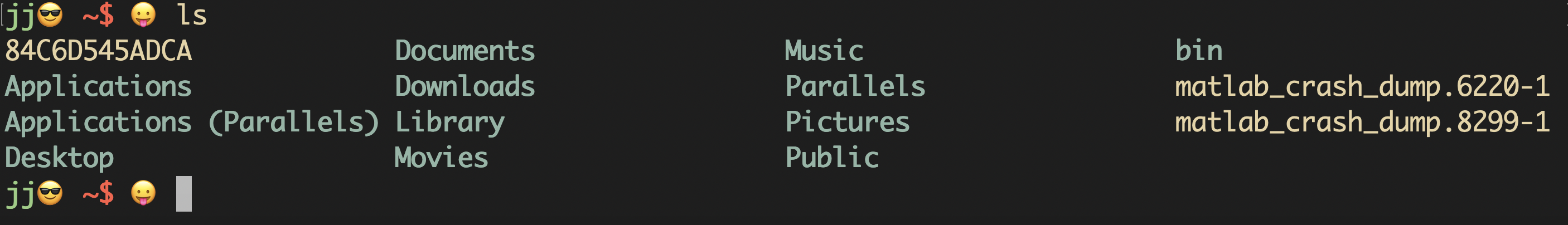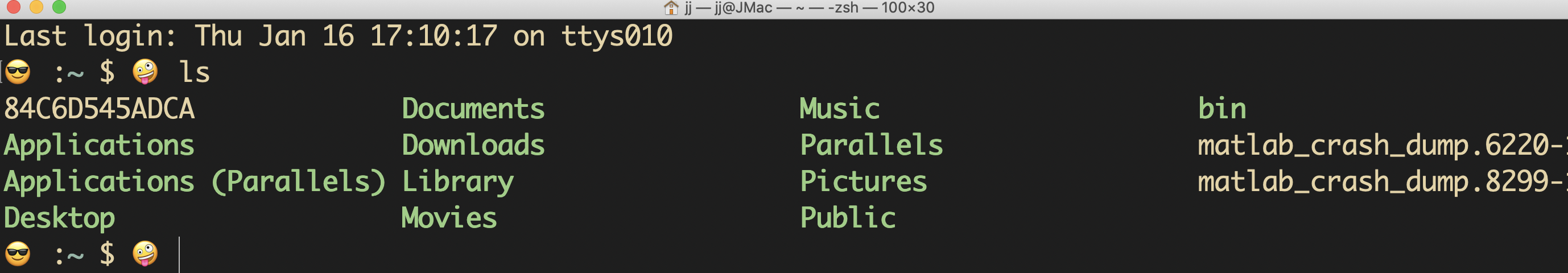# mac终端自定义主题与配色

## 0 写在前面## 1 确定shell

# 下面加了表情
# PS1='$\033[01;36m$\u😎 $\033[01;33m$\h $\033[01;35m$\t $\033[01;31m$\W\$$\033[00m$😛 ' # 去掉时间 # PS1='$\033[01;36m$\u😎 $\033[01;33m$\h$\033[01;31m$\w\\$ $\033[00m$😛 '
# 去掉时间和电脑名字，本人采用
PS1='$\033[01;36m$\u😎 $\033[01;31m$\w\\$$\033[00m$😛 '  然后source .bash_profile生效。贴上一张效果图XD:## 4 关于zsh终端的配置 本部分同样适用于linux，另外注意mac catalina默认是zsh而不是bash，首先切换为zsh脚本环境，具体参考第1节，很多zsh终端配置说需要下载item2，item2是增强版的终端，但是实际上也不需要下载item2 ### 4.1 安装oh-my-zsh 笔者直接用curl安装，注意安装curl之前可能需要安装brew和git等工具，按提示操作即可 sh -c "$(curl -fsSL https://raw.githubusercontent.com/robbyrussell/oh-my-zsh/master/tools/install.sh)"


### 4.2 主题与配色

## based on murilasso
local return_code="%(?..%{$fg[red]%}%? ↵%{$reset_color%})"
local user_host='%{$terminfo[bold]$fg[green]%}%n@%m%{$reset_color%}' local current_dir='%{$terminfo[bold]$fg[blue]%}%~%{$reset_color%}'
local rvm_ruby='%{$fg[red]%}$(rvm_prompt_info || rbenv_prompt_info)%{$reset_color%}' local git_branch='%{$fg[blue]%}$(git_prompt_info)%{$reset_color%}'

# emoj can be found on https://emojipedia.org/grinning-face-with-one-large-and-one-small-eye/
# PROMPT="${user_host}😎 :${current_dir}${rvm_ruby}${git_branch}%b $%b 🤪 " PROMPT="😎 :${current_dir}${rvm_ruby}${git_branch}%b $%b 🤪 " RPS1="${return_code}"

ZSH_THEME_GIT_PROMPT_PREFIX=""
ZSH_THEME_GIT_PROMPT_SUFFIX=""
ZSH_THEME_GIT_PROMPT_DIRTY=" %{$fg[red]%}✗%{$reset_color%}"
ZSH_THEME_GIT_PROMPT_CLEAN=" %{$fg[green]%}✔%{$reset_color%}"©️2019 CSDN 皮肤主题: 黑客帝国 设计师: 上身试试